# heat cond electrical.pdf

17. Jan 2023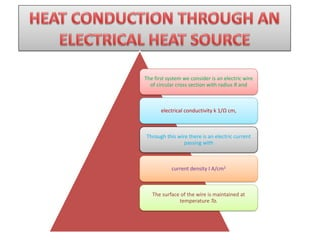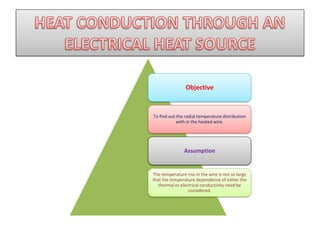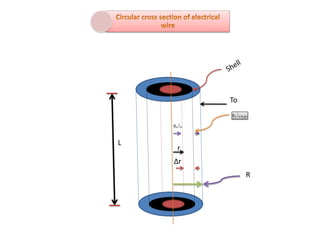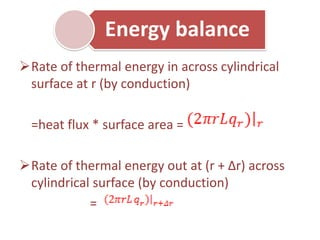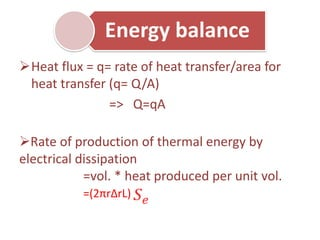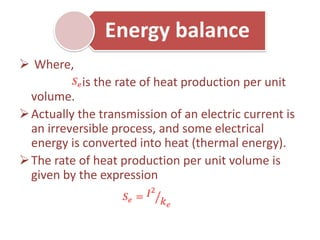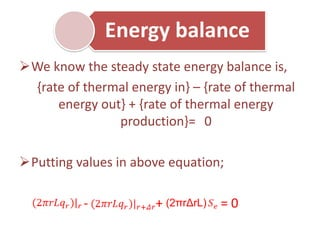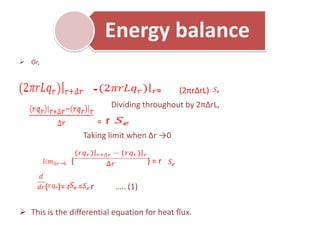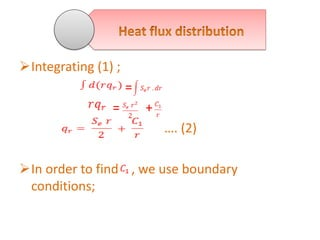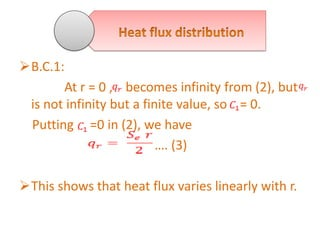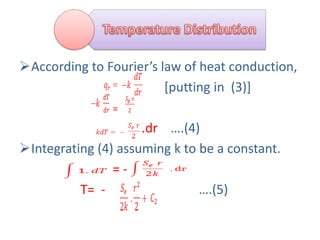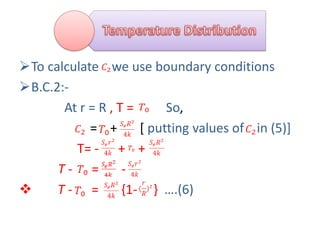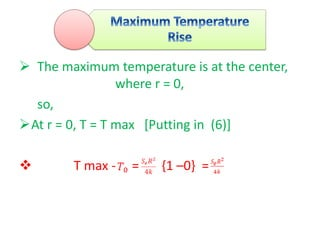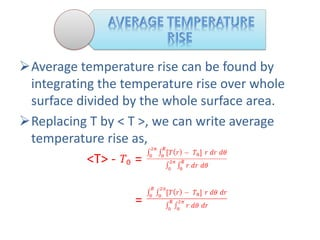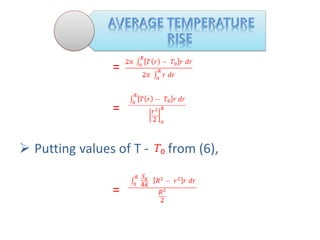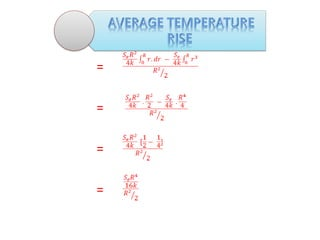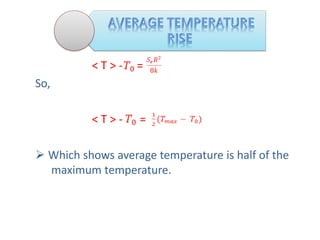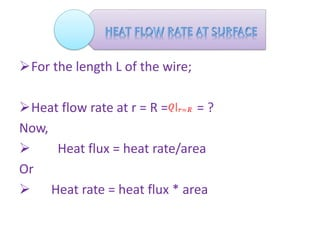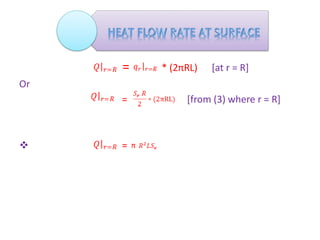1 von 21

### heat cond electrical.pdf

• 1. The first system we consider is an electric wire of circular cross section with radius R and electrical conductivity k 1/Ω cm, Through this wire there is an electric current passing with current density I A/cm2 The surface of the wire is maintained at temperature To.
• 2. Objective To find out the radial temperature distribution with in the heated wire. Assumption The temperature rise in the wire is not so large that the temperature dependence of either the thermal or electrical conductivity need be considered.
• 4. Energy balance ➢Rate of thermal energy in across cylindrical surface at r (by conduction) =heat flux * surface area = ➢Rate of thermal energy out at (r + Δr) across cylindrical surface (by conduction) =
• 5. ➢Heat flux = q= rate of heat transfer/area for heat transfer (q= Q/A) => Q=qA ➢Rate of production of thermal energy by electrical dissipation =vol. * heat produced per unit vol. =(2πrΔrL) Energy balance
• 6. ➢ Where, is the rate of heat production per unit volume. ➢Actually the transmission of an electric current is an irreversible process, and some electrical energy is converted into heat (thermal energy). ➢The rate of heat production per unit volume is given by the expression Energy balance
• 7. ➢We know the steady state energy balance is, {rate of thermal energy in} – {rate of thermal energy out} + {rate of thermal energy production}= 0 ➢Putting values in above equation; - + = 0 (2πrΔrL) Energy balance
• 8. ➢ Or, - = (2πrΔrL) Dividing throughout by 2πΔrL, = r Taking limit when Δr →0 { } = r ( )= r = r ….. (1) ➢ This is the differential equation for heat flux. Energy balance
• 9. ➢Integrating (1) ; = = + …. (2) ➢In order to find , we use boundary conditions;
• 10. ➢B.C.1: At r = 0 , becomes infinity from (2), but is not infinity but a finite value, so = 0. Putting =0 in (2), we have …. (3) ➢This shows that heat flux varies linearly with r.
• 11. ➢According to Fourier’s law of heat conduction, [putting in (3)] = .dr ….(4) ➢Integrating (4) assuming k to be a constant. = - T= - ….(5)
• 12. ➢To calculate we use boundary conditions ➢B.C.2:- At r = R , T = So, = + [ putting values of in (5)] T= - + + T - = - ❖ T - = {1- } ….(6)
• 13. Once heat flux and temperature distribution are known, various quantities about the system may be found. 1. Maximum temperature rise 2. Average temperature rise 3. Heat flow rate at surface
• 14. ➢ The maximum temperature is at the center, where r = 0, so, ➢At r = 0, T = T max [Putting in (6)] ❖ T max - = {1 –0} =
• 15. ➢Average temperature rise can be found by integrating the temperature rise over whole surface divided by the whole surface area. ➢Replacing T by < T >, we can write average temperature rise as, <T> - = =
• 16. = = ➢ Putting values of T - from (6), =
• 18. < T > - = So, < T > - = ➢ Which shows average temperature is half of the maximum temperature.
• 19. ➢For the length L of the wire; ➢Heat flow rate at r = R = = ? Now, ➢ Heat flux = heat rate/area Or ➢ Heat rate = heat flux * area
• 20. = * (2πRL) [at r = R] Or = [from (3) where r = R] ❖ = π